# Machine Learning Building Blocks: Linear Regression

Neural network frameworks, automl solutions and staple numerical libraries, like Scikit-learn and SciPy, have abstracted away much of the logic and math from the implementation of workhorse algorithms. Linear regressions fall into this category. Regressions are fundamental techniques that are often as performant as more complicated models, but we sometimes underestimate them as simply “drawing a line through the data”. This oversimplification leads us to forget that the most basic neural network is infact just a linear regression with no hidden layers, meaning that understanding linear regressions is key to understanding deep learning techniques. Therefore, a deeper understanding of these models can provide better insight into when, where and how to use these techniques, and what (if any) assumptions made by the models impact their business and production use.

To revisit the foundations of linear regression and to learn some of the important functions and processes implicit in modern neural network architecture and training, I will build a simple, applied linear regression model, using basic tensors in Pytorch with support from Autograd. By showing all of the steps, including tensors, linear equations, matrix multiplication and optimisation using gradient descent, I will demonstrate the value of understanding linear regression and how to apply it in your own projects.

## Vectors, Tensors and Linear Equations

Fundamentally, linear equations are the linear combination of elements, often in the form of arrays or matrices. The illustration below highlights this really nicely, whereby there is increased dimensionality from a vector (a 1-D structure) to a matrix (a 2-D structure) to tensors, the latter of which are n-dimensional and can also be interpreted as stacked matrices or arrays. In the following examples we will be using Pytorch’s tensor method to hold the input data for our model, as well as for the weights and bias tensors.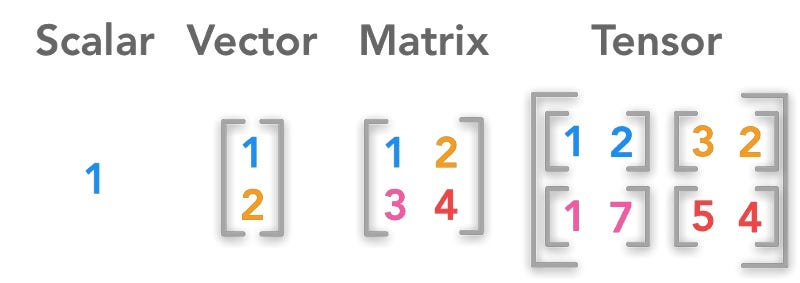A tensor can be used in place of a matrix as input to most models and work well for when your data is highly dimensional or in the case of images where you need to specify pixel intensity as well as colour.

# The Normal Equation

Before we dig into training a model with gradient descent, it is important to know there exists an exact solution for a linear regression. This is called the Normal Equation:

This equation will find the value for θ for features X and target y which will minimize the linear mean square error function for that equation, below:

So if we have some hyper-specifc, lock-down inspired, data from popular pandemic pastimes, such as information on the proofing time, acidity and age of a sourdough starter, we can precisely calculate the matrix of weights that will predict the target flavour and crustiness of the resultant loaf. Note: At this point the author has baked (and eaten) more loaves than held conversations since March 2020.

Using the normal equation, we first calculate the dot product of transpose X (XT) and itself (XTX) then find the inverse of that matrix, (XTX)^-1 . Subsequently we will find the dot product of that inverted matrix and the transpose XT again (XTX)^-1 * XT, before finding the final dot product of the resultant matrix from that calculation with the target features y, (XTX)^-1 * XT y. The result of this calculation will be a matrix of weights we can use to predict the target features for new batches of sourdough. There is an important caveat here, which is that these represent the weights which will return the minimal loss. This does not mean they perfectly predict every new target, just that the linear equation described by these values fits the data as well as is mathematically possible. In other words, they are the global minima. Below is a one linear for calculating finding the solution to the Normal equation using Numpy linalg, T and dot methods. In the below example I have used the afore-mentioned sourdough data, which I will cover later.

So if we have an equation for finding the best possible values for any linear regression, why would we need to solve with any other methodology? The normal equation deals well with small data, it can even be reasonably computed by hand for small enough sets, but it does not scale well with feature size. While matrix multiplication is computationally simple, the matrix inversion step is inefficient, somewhere between O(n²)-O(n³). So in the worst case, doubling the features would be eight times as computationally expensive, meaning that with hundreds of thousands of features this operation really starts to chug. Therefore, to model more complex linear regressions we need a different tool; we need gradient descent.

We are going to start with a very simple example in which we can also introduce Autograd and its autodifferentiation functionalities. The first thing we are going to do is instantiate some tensors with values to solve a linear equation like Y = W * X + B: Where the values of X, W and B are given as 1, 2 and 3 respectively.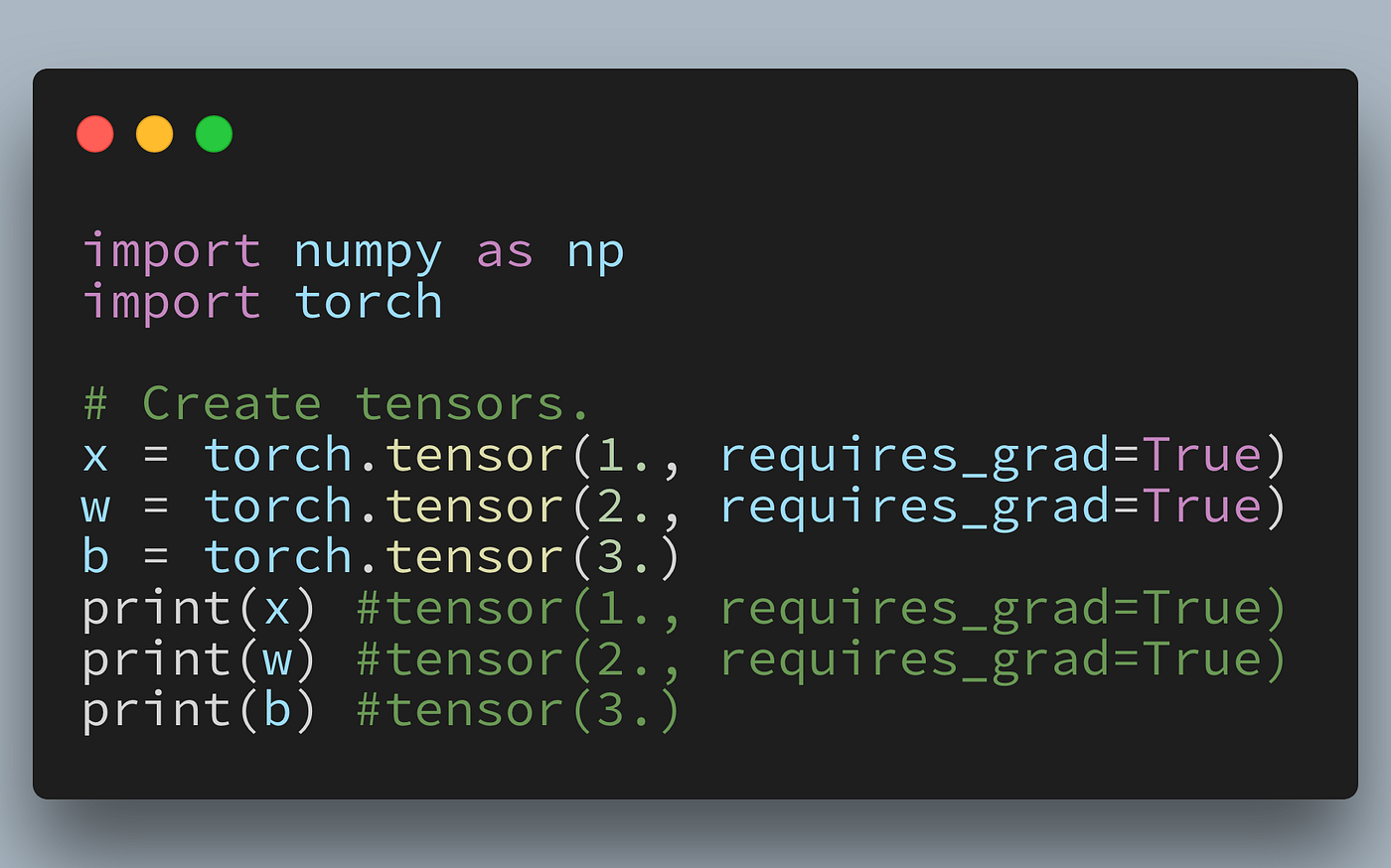Torch has a tensor and tensor manipulation API that is highly inspired by Numpy. You can effectively translate directly, or almost directly, between the two libraries.

We have now created the W, X and B variables, and declared `requires_grad=True` for X and W. This creates a computational graph for W and X which tracks the operation history for these variables and performs automagical gradient calculations. Autodifferentiation will be important later as fast gradient calculations will make the gradient descent optimisation much quicker. Below is an example of the computational graph for the multiplication of two variables, x and y. You can see that the gradient of the variable x is automatically calculated as 2 when there is a call to backward method from the output variable, z.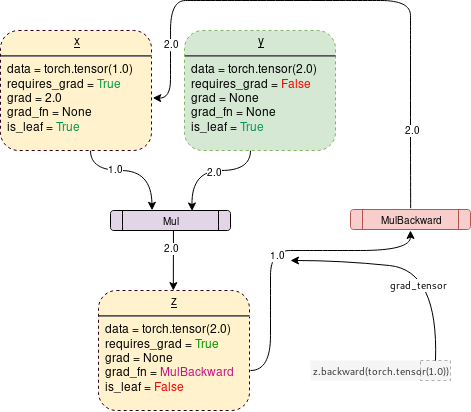The computational graph that is created for all of the elements in the multiplication of 2*1 where the gradient was required.

To solve the linear equation above for our Y, all we need to do is multiply our features (X) by our weights (W), then add the bias (B). This gives us the value for Y as 5. Now we can illustrate what we are using the autodifferentiation capabilities of PyTorch/Autograd for. Given that we know the product of these tensors is 5, we know that the product of W or X and its gradient, the slope of the equation at that point, plus the intercept B, will give us 5. We can prove this below.

To check: x = 1, its gradient is 2 so X * X.grad + B = 5. Keep this in mind, this will come in handy later when we are using this feature to minimize a loss function to converge another linear regression model. At this point, it is worth emphasising that the equation for a linear regression is extendable past single variables and as stated early is often computed for vectors and matrices. The below diagram shows the same linear equation of Y = W*X + B for matrices instead of single point values.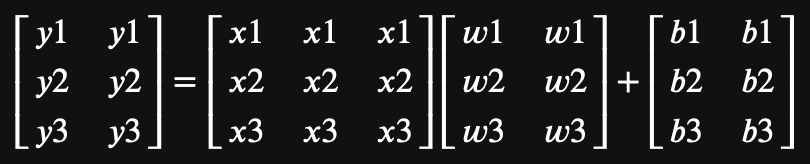This is the same general equation of the linear equation y = W*X + B, only we are using matrices instead of the single values about. All of the same operation occur, multiplication, addition, only the structure of the data is different.

## Linear Regression Data

As I am writing this in March 2021, and we are still very much in lockdown, we are going to try and predict the flavour (subjective) and degree of crustiness (also subjective) of my homemade sourdough to the measured proofing time, acidity and age of my starter.

I have used numpy to create the arrays, below, as this api is probably most familiar to people. I will then convert them to torch tensors to show how easy it is to pull things into the Pytorch environment. Note, this could have been done from the start by creating the inputs and targets with torch.tensor().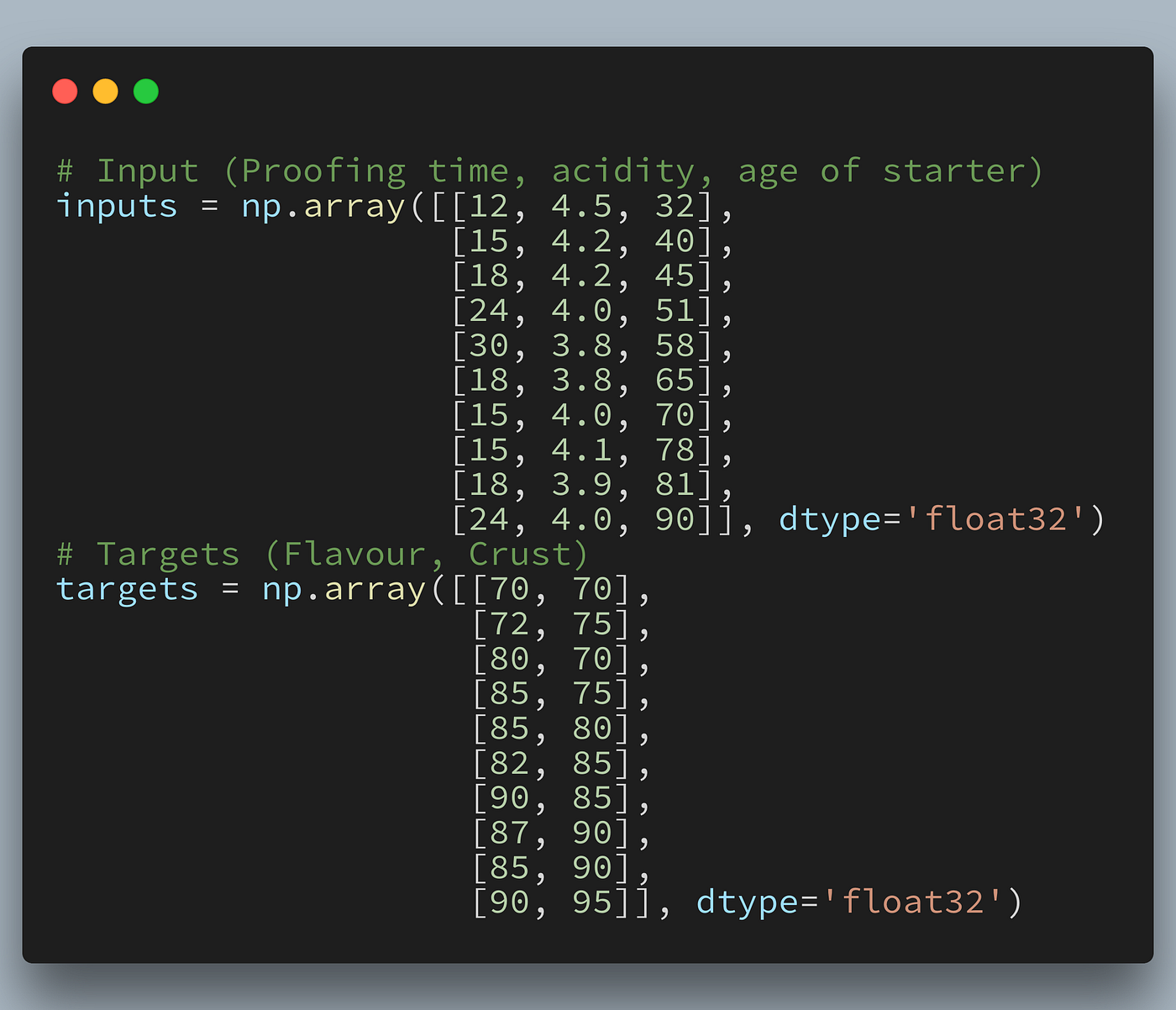Here we are using the Numpy array method to create the input and target data, torch.Tensor is also an option for this exact same thing. The translation from package to package is seemless too as the Pytorch team took inspiration for their api from Numpy.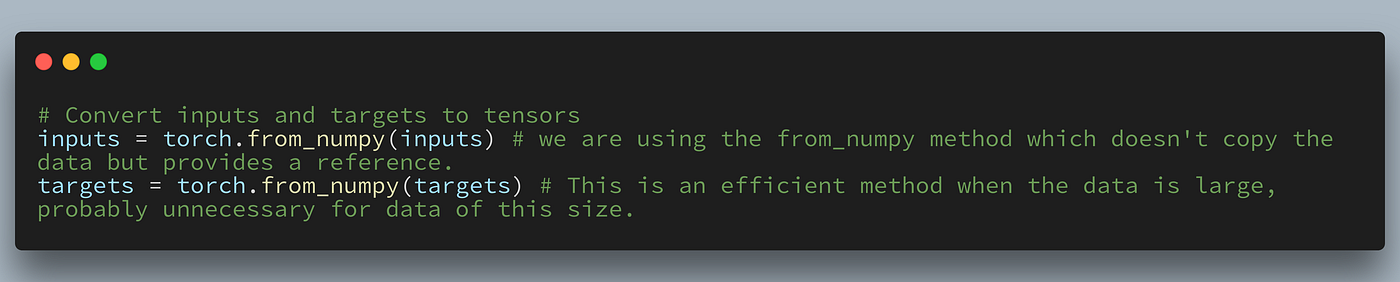Converting from Numpy arrays to Torch tensors is trivial with the from_numpy() method.

Because this data is very small, the Normal Equation would be appropriate, however we will be building a gradient descent model to illustrate how to solve using this method for larger datasets. In an ideal situation, we would multiply our inputs, X, by some known weights, W, and add a known bias, B. This will return our target values of Y for the correct values of W and B. Now, we are unlikely to guess the correct values of W and B on the first guess, but there are a few ways forward. We could grid or random search a matrix of values to brute force this, but this is not a very sophisticated method and will not necessarily find the minima for a latent space if that area is outside the defined search area. So we will first create random weights and biases, again requiring grad for autodifferentiation. The weights are in the shape 2x3, we will multiply the inputs by the transpose of this matrix to obtain the desired shape of the target tensor. With that in mind we can create our “model” for this problem which will be the matrix X, multiplied by the transpose of the weights (W.t()) + the bias B. It is also important to understand how far each prediction is from the ground truth so we also need to define a loss function to measure the degree of error. We will use the metric of mean square error, MSE, which is defined below as the normalized square of the error.

So with our model defined and a function for calculating our loss, we can now iteratively start to find our loss function minimising weights to solve this regression. To do this we will create a training loop for a given number of epochs. In each epoch, we will create predictions from our input using the model we just defined and randomly instantiated weights, W, and a bias, B. For each set of predictions we will calculate the loss, then back propagate this loss with the autograd call backward(). We will then update the weights and biases by subtracting their gradient multiplied by some tiny value. We subtract because this will step the loss function down the gradient, toward an area of lower loss. The gradient will then be cleared so we don’t accumulate them and the next epoch will start with these updated weights and biases and the training loop will continue this cycle of: 1) Prediction, 2) loss calculation, 3) backward propagation, 4) model updating for the predetermined number of epochs.

We end up with two tensors, one for the weights and one for the biases, that minimize the loss function for the linear equation we are using.

We could now solve for a new input of observed measurements in to the original linear equation and predict the output of the result loaf. This method of linear regression should ideally result in the same amount of loss as the Normal Equation so the two methods are “equal” in their performance as measured by the accuracy of the result. To iterate, you will want to select one method over the other depending entirely on the size of the data you are training on. As your training data approaches the limits of your machine’s memory and the matrix inversion step becomes a rate limiting element you want to start considering using gradient descent.

# Using the NN Built-in Methods of PyTorch

We have now covered the building blocks of a linear regression, from the normal equation to how to use autodifferentiation tools to optimize weights and biases, and we defined our own loss metrics and fine tuned the LR model to reasonably predict the outcome for a set of inputs. The next step in complexity is to recreate this model using a pre-built framework, PyTorch, and build the model as a single layer neural network with a linear transformation. By doing this, we can also take advantage of pre-built loss functions and optimizers, which will drastically simplify our training loop. Below is the code to create the exact same model using PyTorch nn.Module and nn.Linear for the forward pass. This kind of solution is simpler to write as we don’t need to define every function ourselves. It also has the added benefit of multiple pre-written optimizers if we want to do something more intricate than gradient descent like using an Adam optimizer, or different loss functions and even changing up the learning rate dynamically by adding learning rate schedulers. Importantly, now that we have created each individual step from scratch we have a better understanding of what each function is doing and why.

This results in approximately the exact same loss, model weights and biases as our hand crafted model and the Normal Equation variation but as just stated comes with a few added benefits we can take advantage of because we are using such a robust framework. It’s definitely worth noting that the choice of PyTorch for this project was also intentional as the ability to use GPU’s is baked into the framework. By calling for the available device, cuda:0 if available, otherwise CPU, the inputs, targets and model can all be loaded into GPU memory and hardware accelerated. This is becoming more important as data becomes larger and converging these models becomes a more intensive job.

--

--

--

## More from Richard Bownes

BBC Data Scientist

Love podcasts or audiobooks? Learn on the go with our new app.

## N-step TD Method## Task 1 :- How to Run Machine Learning Models on Docker Container.## Pytorch FAQ Code Snippets for Udacity Deep Learning Scholarship Challenge with Facebook## Machine Learning Model inside container using Docker## Build and Deploy Your Own Machine Learning Web Application by Streamlit and Heroku## Why transfer learning works or fails?## fast.ai 2020 — Lesson 7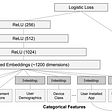## How I built a Trade extractor that is still the best self-learning indicator in the Forex…## Richard Bownes

BBC Data Scientist

## Data Science Clustering Countries with K-means Clustering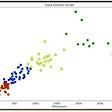## K-Nearest Neighbors Classification## Regularised Linear Regression.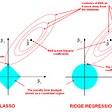## Linear Classification in Machine Learning